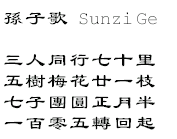Oystein Ore mentions the following puzzle from Brahma-Sphuta-Siddhanta (Brahma’s Correct System) by Brahmagupta :

An old woman goes to market and a horse steps on her basket and crashes the eggs. The rider offers to pay for the damages and asks her how many eggs she had brought. She does not remember the exact number, but when she had taken them out two at a time, there was one egg left. The same happened when she picked them out three, four, five, and six at a time, but when she took them seven at a time they came out even. What is the smallest number of eggs she could have had?

Here’s a similar problem from “Advanced Number Theory” by Harvey Cohn (( always, i wonder how one might ‘discreetly request’ these remainders… )) :

Exercise 5 : In a game for guessing a person’s age x, one discreetly requests three remainders : r1 when x is divided by 3, r2 when x is divided by 4, and r3 when x is divided by 5. Then x=40 r1 + 45 r2 + 36 r3 modulo 60.

Clearly, these problems are all examples of the Chinese Remainder Theorem.Chinese because one of the first such problems was posed by Sunzi [Sun Tsu] (4th century AD)
in the book Sunzi Suanjing. (( according to ChinaPage the answer is contained in the song on the left hand side. ))

There are certain things whose number is unknown.
Repeatedly divided by 3, the remainder is 2;
by 5 the remainder is 3;
and by 7 the remainder is 2.
What will be the number?

The Chinese Remainder Theorem asserts that when $N=n_1n_2 \ldots n_k$ with the $n_i$ pairwise coprime, then there is an isomorphism of abelian groups $\mathbb{Z}/N \mathbb{Z} \simeq \mathbb{Z}/n_1 \mathbb{Z} \times \mathbb{Z}/n_2 \mathbb{Z} \times \ldots \times \mathbb{Z}/n_k \mathbb{Z}$. Equivalently, given coprime numbers $n_i$ one cal always solve the system of congruence identities

$\begin{cases} x \equiv a_1~(\text{mod}~n_1) \\ x \equiv a_2~(\text{mod}~n_2) \\ \vdots \\ x \equiv a_k~(\text{mod}~n_k) \end{cases}$

and all integer solutions are congruent to each other modulo $N=n_1 n_2 \ldots n_k$.

We will need this classical result to prove that
$\mathbb{Q}/\mathbb{Z} \simeq \mathcal{A}/\mathcal{R}$
where (as last time) $\mathcal{A}$ is the additive group of all adeles and where $\mathcal{R}$ is the subgroup $\prod_p \mathbb{Z}_p$ (i’ll drop all ‘hats’ from now on, so the p-adic numbers are $\mathbb{Q}_p = \hat{\mathbb{Q}}_p$ and the p-adic integers are denoted $\mathbb{Z}_p = \hat{\mathbb{Z}}_p$).

As we will have to do calculations with p-adic numbers, it is best to have them in a canonical form using digits. A system of digits $\mathbf{D}$ of $\mathbb{Q}_p$ consists of zero and a system of representatives of units of $\mathbb{Z}_p^*$ modulo $p \mathbb{Z}_p$. The most obvious choice of digits is $\mathbf{D} = { 0,1,2,\ldots,p-1 }$ which we will use today. (( later we will use another system of digits, the Teichmuller digits using $p-1$-th root of unities in $\mathbb{Q}_p$. )) Fixing a set of digits $\mathbf{D}$, any p-adic number $a_p \in \mathbb{Q}_p$ can be expressed uniquely in the form

$a_p = \sum_{n=deg(a_p)}^{\infty} a_p(n) p^n$ with all ‘coefficients’ $a_p(n) \in \mathbf{D}$ and $deg(a_p)$ being the lowest p-power occurring in the description of $a_p$.

Recall that an adele is an element $a = (a_2,a_3,a_5,\ldots ) \in \prod_p \mathbb{Q}_p$ such that for almost all prime numbers p $a_p \in \mathbb{Z}_p$ (that is $deg(a_p) \geq 0$). Denote the finite set of primes p such that $deg(a_p) < 0$ with $\mathbf{P} = { p_1,\ldots,p_k }$ and let $d_i = -deg(a_{p_i})$. Then, with $N=p_1^{d_1}p_2^{d_2} \ldots p_k^{d_k}$ we have that $N a_{p_i} \in \mathbb{Z}_{p_i}$. Observe that for all other prime numbers $q \notin \mathbf{P}$ we have $~(N,q)=1$ and therefore $N$ is invertible in $\mathbb{Z}_q$.

Also $N = p_i^{d_i} K_i$ with $K_i \in \mathbb{Z}_{p_i}^*$. With respect to the system of digits $\mathbf{D} = { 0,1,\ldots,p-1 }$ we have

$N a_{p_i} = \underbrace{K_i \sum_{j=0}^{d_i-1} a_{p_i}(-d_i+j) p_i^j}_{= \alpha_i} + K_i \sum_{j \geq d_i} a_{p_i}(-d_i+j)p_i^j \in \mathbb{Z}_{p_i}$

Note that $\alpha_i \in \mathbb{Z}$ and the Chinese Remainder Theorem asserts the existence of an integral solution $M \in \mathbb{Z}$ to the system of congruences

$\begin{cases} M \equiv \alpha_1~\text{modulo}~p_1^{d_1} \\ M \equiv \alpha_2~\text{modulo}~p_2^{d_2} \\ \vdots \\ M \equiv \alpha_k~\text{modulo}~p_k^{d_k} \end{cases}$

But then, for all $1 \leq i \leq k$ we have $N a_{p_i} – M = p_i^{d_i} \sum_{j=0}^{\infty} b_i(j) p^j$ (with the $b_i(j) \in \mathbf{D}$) and therefore

$a_{p_i} – \frac{M}{N} = \frac{1}{K_i} \sum_{j=0}^{\infty} b_i(j) p^j \in \mathbb{Z}_{p_i}$

But for all other primes $q \notin \mathbf{P}$ we have that $\alpha_q \in \mathbb{Z}_q$ and that $N \in \mathbb{Z}_q^*$ whence for those primes we also have that $\alpha_q – \frac{M}{N} \in \mathbb{Z}_q$.

Finally, observe that the diagonal embedding of $\mathbb{Q}$ in $\prod_p \mathbb{Q}_p$ lies entirely in the adele ring $\mathcal{A}$ as a rational number has only finitely many primes appearing in its denominator. Hence, identifying $\mathbb{Q} \subset \mathcal{A}$ via the diagonal embedding we can rephrase the above as

$a – \frac{M}{N} \in \mathcal{R} = \prod_p \mathbb{Z}_p$

That is, any adele class $\mathcal{A}/\mathcal{R}$ has as a representant a rational number. But then, $\mathcal{A}/\mathcal{R} \simeq \mathbb{Q}/\mathbb{Z}$ which will allow us to give an adelic version of the Bost-Connes algebra!

Btw. there were 301 eggs.

Published in featured

## One Comment

This site uses Akismet to reduce spam. Learn how your comment data is processed.